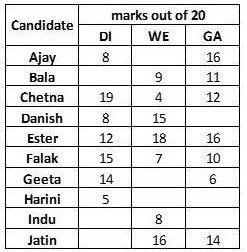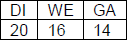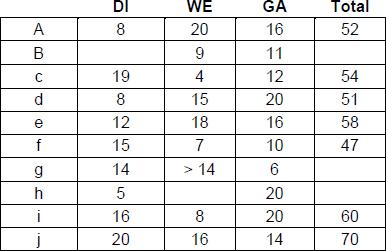### CAT 2019 - Slot 1 - Logical Reasoning and Data Interpretation - A company administers a written test comprising of three sections

##### A company administers a written test comprising of three sections of 20 marks each – Data Interpretation (DI), Written English (WE) and General Awareness (GA), for recruitment. A composite score for a candidate (out of 80) is calculated by doubling her marks in DI and adding it to the sum of her marks in the other two sections. Candidates who score less than 70% marks in two or more sections are disqualified. From among the rest, the four with the highest composite scores are recruited. If four or less candidates qualify, all who qualify are recruited. Ten candidates appeared for the written test. Their marks in the test are given in the table below. Some marks in the table are missing, but the following facts are known: 1. No two candidates had the same composite score. 2. Ajay was the unique highest scorer in WE. 3. Among the four recruited, Geeta had the lowest composite score. 4. Indu was recruited. 5. Danish, Harini, and Indu had scored the same marks the in GA. 6. Indu and Jatin both scored 100% in exactly one section and Jatin’s composite score was 10 more than Indu’s.Q. 1: Which of the following statements MUST be true?
1. Jatin’s composite score was more than that of Danish.
2. Indu scored less than Chetna in DI.
3. Jatin scored more than Indu in GA.
1. Only 2
2. Only 1
3. Both 1 and 2
4. Both 2 and 3

##### Q. 2: Which of the following statements MUST be FALSE?1. Chetna scored more than Bala in DI2. Harini’s composite score was less than that of Falak3. Bala’s composite score was less than that of Ester4. Bala scored same as Jatin in DI

Q. 3: If all the candidates except Ajay and Danish had different marks in DI, and Bala’s composite score was less than Chetna’s composite score, then what is the maximum marks that Bala could have scored in DI?

Q. 4: If all the candidates scored different marks in WE then what is the maximum marks that Harini could have scored in WE?

Given, Indu was recruited and Indu scored 100% in exactly one section. Jatin scored 100% in exactly one section

=> Jatin’s scored areComposite score = 20 x + 2 + 16 + 14 = 70

Indu’s score is 70 – 10 =60

If Indu scores 20 in DI, Indus’s score in GA = 60 – 40 – 8 = 12 In this case, Indu will not quality Hence, Indu scored 20 in GA.

score in

Dl = (60 – 20 – 8) / 2 = 32 / 2 = 16

Danish, Harini and Indu scored 20 in GA Score of Danish is 2(8) + 15 + 20 = 51

Hence, Score of Ajay is 2(8) + 20 + 16 = 52

(As Ajay scores either 19 or 20 in DI, the composite score cannot be 51)Question 1:

(Jatin’s composite score was more than that of Danish) and (Indu scored less than Chetan in DI).

Ans : Both 1 and 2

Question 2:

If Bala scores 20 in DI, Score = 2(20) + 9 + 11 = 60, which is the same as that of Indu. Not possible

Hence, Bala scored same as Jatin in DI must be false. Ans : Bala scored same as Jatin in DI

Question 3:

Ans: 13

Question 4:

Ans: 14

Inspiring Education… Assuring Success!!

Ghatkopar | Borivali | Andheri | Online